# Difference between revisions of "ImuCalibration"

## Theory

Accelerometer and Magnetometer calibration is critical to AHRS performance and can be performed using no special hardware. For the magnetometer, it is very important that the calibration be performed in the fully assembled vehicle, with all systems powered on. This is called hard-iron calibration and will allow us to compensate for any constant parasitic magnetic fields generated by the vehicle. The calibration process consists of finding a set of neutrals and scale factors for each sensor, such as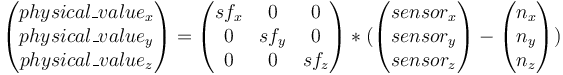$\begin{pmatrix}physical\_value_x\\physical\_value_y\\physical\_value_z\end{pmatrix} = \begin{pmatrix}sf_x&0&0\\0&sf_y&0\\0&0&sf_z\end{pmatrix} * (\begin{pmatrix}sensor_x\\sensor_y\\sensor_z\end{pmatrix}-\begin{pmatrix}n_x\\n_y\\n_z\end{pmatrix})$

The principle of the calibration is the following: an accelerometer, on a vehicle at rest, measures a constant vector (the opposite of gravity) in the earth frame, expressed in the vehicle frame.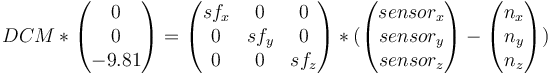$DCM * \begin{pmatrix}0\\0\\-9.81\end{pmatrix} = \begin{pmatrix}sf_x&0&0\\0&sf_y&0\\0&0&sf_z\end{pmatrix} * (\begin{pmatrix}sensor_x\\sensor_y\\sensor_z\end{pmatrix}-\begin{pmatrix}n_x\\n_y\\n_z\end{pmatrix})$

DCM is a rotation matrix that converts between earth frame and body frame. It will change when we change the orientation of the vehicle. Nevertheless, a rotation conserves the norm of a vector. We can thus obtain the following scalar equation that doesn't depend on the vehicle orientation :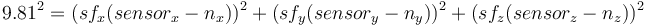$9.81^2 = ( sf_x(sensor_x-n_x) )^2 + (sf_y(sensor_y-n_y) )^2 + (sf_z(sensor_z-n_z) )^2$

We can then record an important number of measurements in different orientations and find the set of scale factors and neutrals giving the norm closest to 9.81

### How It Works

• It first makes an initial guess using min and max, i.e. for each axis
• neutral = 0.5 * (max + min)
• sensitivity = 0.5*(max-min)
• It then uses a data fitting algorithm to optimize the initial guess.

Screenshot of Scilab version.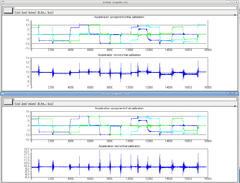## Calibration Script Installation

Paparazzi comes with a Python script to calibrate the accelerometers and magnetometer. The application can be found in the Paparazzi directory under:

sw/tools/calibration/calibrate.py


For the application to work, however, you need additional Python libraries. If you already have the package paparazzi-dev installed, the needed libraries were already installed as dependencies. If this is not the case you need to install python-scipy and python-matplotlib. This can be done via Synaptic Package Manager or via the command-line of Ubuntu.

$sudo apt-get install python-scipy$ sudo apt-get install python-matplotlib


## How to Calibrate Your IMU

• Flash the board with your normal AP firmware (if it is not already on it.)
• Switch to the "raw sensors" telemetry mode via GCS->Settings->Telemetry and launch "server" to record a log.
• Move your IMU/airframe into different positions to record relevant measurements for each axis.
• It is important that you get the min/max sensor values on each axis!
• Stop the server so it will write the log file (to var/logs).
• Run the Python script on it to get your calibration coefficients.
• If you have data from more than one aircraft in your logfile, you will need to specify the aircraft id via the --id option.
It can be found in the Paparazzi Center upper left side. (first comes A/C name, and then id value)

### Calibrating the Accelerometers

To calibrate the accelerometers turn the IMU on all six sides of the cube:

1. upright,
2. inverted,
3. on the nose,
4. on the tail,
5. on the right side,
6. on the left side.

You can also take some measurements banking 45 degrees.
Try to get a homogeneous distribution of your measurements. It is better to let the aircraft rest while measuring.

Then stop the server so it will write the log file and run the Python script on it to get your calibration coefficients:

sw/tools/calibration/calibrate.py -s ACCEL <path_to_data_file var/logs/xxxxxxx.data>


### Calibrating the Magnetometer

This is the most crucial part for the magnetometer calibration: you really need to get the min/max on each axis, so align the magnetometer axes along the local magnetic field vector (not just take the same orientations as when calibrating the accelerometer.)

It is usually convenient to plot the values to see when you get the maximum on each axis. The inclination and declination of the magnetic field where you live can be looked up here.

sw/tools/calibration/calibrate.py -s MAG <path_to_data_file var/logs/xxxxxxx.data>


The magnetic field changes depending on where you are in the world, because of this you have to set the local magnetic field. Or if you are using the older euler filter recalibrate your magnetometer to fly somewhere else.

### Calibrating the Gyroscopes

To calibrate the gyros you need a turntable....

## Finding and Checking Signs

For supported IMUs, the correct default signs are already defined in the code.

If using a new IMU or sign for yours are not in the code yet, here is the way to find them.

We're calibrating everything relative to the IMU frame - Paparazzi has a parameter to define the orientation of the IMU with respect to the body of the vehicle that we'll use later, once you'll have decided of a good mechanical mounting.

Paparazzi uses North East Down (NED) frame, that is positive x is pointing to the front, positive y to the right and positive z down.

### Accelerometer:

An accelerometer measures the non gravitational acceleration, that is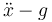$\ddot{x} - g$.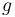$g$ is pointing down, so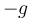$-g$ is pointing up. So stop moving, disregard earth rotation and you'll measure$-g$.

• When your IMU is level you should see x=0 y=0 z=-9.81
• When pitching up -g is aligning with x, so you should see x>0, y=0 and z<0
• When banking left -g is aligning with y, so you should see x=0, y>0 and z<0

### Magnetometer:

A magnetometer measures the Earth's magnetic field. In the northern hemisphere, this points north and down and in the Southern hemisphere north and up.

Thus in the northern hemisphere:

• When you align your IMU with the direction of north, you should see x>0, y=0, z>0.
• When pitching the IMU down, the magnetic vector is aligning with x, so x should increase and z should decrease to zero.
• If yawing your IMU to the left, the magnetic vector is aligning with y, so y should be positive, x should decrease to zero and z stay positive.

And in the southern hemisphere:

• When you align your IMU with the direction of north, you should see x>0, y=0, z<0
• When pitching the IMU up, the magnetic vector is aligning with x, so x should increase and z should increase towards zero.
• If yawing your IMU to the left, the magnetic vector is aligning with y, so y should be positive, x should decrease to zero and z stay negative.

### Gyrometer:

You need some turntable to calibrate the scale factors of your gyros. For signs, the definition of the frame gives the following properties:

• When rolling right,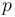$p$ should be positive.
• When pitching up,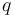$q$ should be positive.
• When yawing to the right,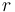$r$ should be positive.

### Verification:

Switch to AHRS telemetry mode and look for the fields that are prefixed with imu_

• Bank right should give positive phi
• Pitch up should give positive theta
• Yaw right should give increasing psi
• The value you'll see after letting the IMU rest will end up being the "measure" (that is accelerometer and magnetometer.) If those are wrong, the problem is in the calibration of your sensors.
• The values you get while moving the IMU are influenced by the gyros. If what you see is the value going crazy when you move and then stabilizing to something good after you stop moving, the problem is in your gyros.

## Body to IMU Rotation

Provision is made within the software to physically locate supported IMUs in orientations with respect to the aircraft/rotorcraft frame which suit available mounting options.

• The in-line, parallel mount example:

Configured as:

 File: conf/airframes/myplane.xml  

As noted further above, calibration and sign verification procedures are carried out relative to the IMU frame, hence defines should be left at zero until they are completed.

• The positive 90 degree offset, parallel mount example:

Configured as:

 File: conf/airframes/myplane.xml  
• Another variation might include turning the IMU upside-down in the parallel plane:

Configured as:

 File: conf/airframes/myplane.xml  

### In Flight Tuning

• Switch to AHRS telemetry mode and look for the fields that are prefixed with imu_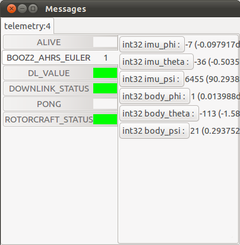90 degree positive offset example

• Open a Real_time plotter from the paparazzi console tools tab and drag int32 body_phi and int32 body_theta into the plotter box.
• Hover rotorcraft (preferably indoors) to record data.
• Copy the (best fit) recorded values into the airframe BODY_TO_IMU defines:
 File: conf/airframes/myplane.xml  
• Ignore 'on the ground' resting values as depicted in the above image. They will change once the vehicle is in flight. The aim is to get the 'in flight' values of "int32 body_phi" & "int32 body_theta" as close to zero as possible.